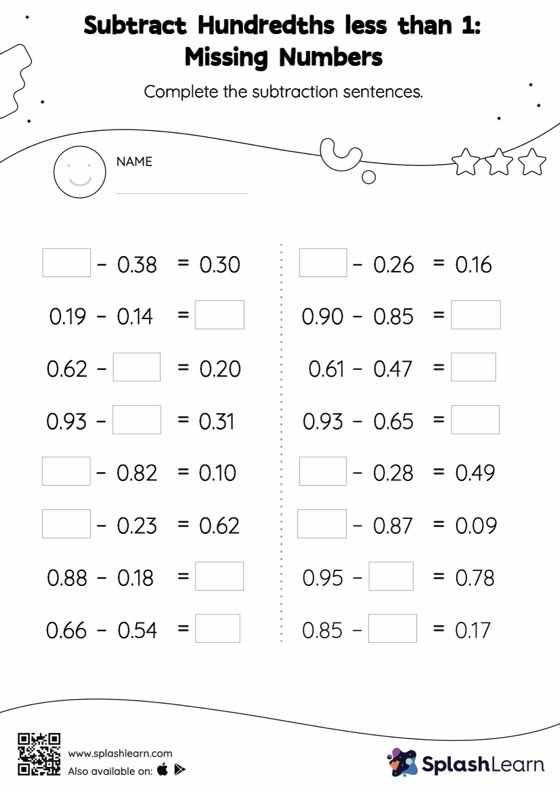# Subtract Hundredths less than 1: Missing Numbers Worksheet

Home > Subtract Hundredths less than 1: Missing NumbersCan your young mathematician subtract hundredths less than 1? That's exactly what they do here. As the worksheet uses a horizontal format, it allows for more creativity when it comes to how the student solves the problem. In contrast, in the vertical format, students usually employ the standard method to solve.